# Help re-engineering indicator127

I found an indicator a couple of years again. I don't know whom wrote it but it had this header at top so credit is due here

```//+------------------------------------------------------------------+
//|                        Copyright 2016, MetaQuotes Software Corp. |
//|                                   https://M2P_Design@hotmail.com |
//+------------------------------------------------------------------+

I've reworked the code but have never liked how it first applies itself to a chart and then how quickly it slows down, bogs and the crashes the terminal. Which is a pity cause it is a great little indicator

Here is the code

```//+------------------------------------------------------------------+
//|                               Master Indicator Template v3.0 mq4 |
//|                                                forexbob@live.com |
//+------------------------------------------------------------------+
#property version   "3.0"
#property strict
#property indicator_chart_window
//+------------------------------------------------------------------+
//|                                                                  |
//+------------------------------------------------------------------+
//--- indicator settings
#property indicator_separate_window
#property indicator_buffers 4
#property indicator_color1 Green
#property indicator_color2 LimeGreen
#property indicator_color3 Orange
#property indicator_color4 Red
//--- indicator levels
#property indicator_level1     40.0
#property indicator_level2     50.0
#property indicator_level3     60.0
#property indicator_levelcolor clrDimGray
#property indicator_levelstyle STYLE_DOT

//--- input parameter
input int    InpPeriod=89;

//+------------------------------------------------------------------+
//| Custom indicator initialization function                         |
//+------------------------------------------------------------------+
int OnInit()
{
string short_name;

//--- 3 additional buffer used for counting.
IndicatorBuffers(8);
SetIndexBuffer(0,BullPowerIndicator);
SetIndexBuffer(1,BullSentimentIndicator);
SetIndexBuffer(2,BearSentimentIndicator);
SetIndexBuffer(3,BearPowerIndicator);

//--- Accuracy of Indicator
IndicatorDigits(1);

//--- drawing style
SetIndexStyle(0,DRAW_LINE,STYLE_SOLID,2);
SetIndexStyle(1,DRAW_LINE,STYLE_SOLID,2);
SetIndexStyle(2,DRAW_LINE,STYLE_SOLID,2);
SetIndexStyle(3,DRAW_LINE,STYLE_SOLID,2);

//--- name for DataWindow and indicator subwindow label
short_name="Bobs Strength Meter("+IntegerToString(InpPeriod)+")";

//--- name for DataWindow and indicator subwindow label
short_name="Bobs Strength Meter("+IntegerToString(InpPeriod)+")";

SetIndexLabel(1,"Sell Sentiment");
SetIndexLabel(3,"Sell Power");

//--- check for input parameter
if(InpPeriod<=0)
{
Print("Wrong input parameter Delta = ",InpPeriod);
return(INIT_FAILED);
}
//---
return(INIT_SUCCEEDED);
}
//+------------------------------------------------------------------+
//| Custom indicator deinitialization function                       |
//+------------------------------------------------------------------+
int deinit()
{

return(0);
}
//+------------------------------------------------------------------+
//| Custom indicator iteration function                              |
//+------------------------------------------------------------------+
int OnCalculate(const int rates_total,
const int prev_calculated,
const datetime &time[],
const double &open[],
const double &high[],
const double &low[],
const double &close[],
const long &tick_volume[],
const long &volume[],
{
ArrayResize(BullSentiment,Bars+InpPeriod);
ArrayResize(BearSentiment,Bars+InpPeriod);
ArrayResize(BullPower,Bars+InpPeriod);
ArrayResize(BearPower,Bars+InpPeriod);
ArrayResize(BullSentimentIndicator,Bars+1);
ArrayResize(BearSentimentIndicator,Bars+1);
ArrayResize(BullPowerIndicator,Bars+1);
ArrayResize(BearPowerIndicator,Bars+1);

for(int i=0; i<rates_total; i++)
{
Sentiment(i);
Power(i);

double Bull1=0,Bear1=0;
double Bull2=0,Bear2=0;

for(int cnt=i; cnt<(i+InpPeriod); cnt++)
{
Bull1=Bull1+BullSentiment[cnt];
Bear1=Bear1+BearSentiment[cnt];
Bull2=Bull2+BullPower[cnt];
Bear2=Bear2+BearPower[cnt];
}

double TotalPips=Bull2+Bear2;

BullSentimentIndicator[i]=Bull1/InpPeriod;
BearSentimentIndicator[i]=Bear1/InpPeriod;
BullPowerIndicator[i] = MathRound((Bull2/TotalPips)*100);
BearPowerIndicator[i] = MathRound((Bear2/TotalPips)*100);
}
return(rates_total);
}
//+------------------------------------------------------------------+

//+----------------------------------------+
//  Sentiment                              |
//+----------------------------------------+
void Sentiment(int i)
//+----------------------------------------+
{
double Percent=0;
double Bulls=0,Bears=0;
double Length0=(iHigh(Symbol(),0,i)-iLow(Symbol(),0,i));

double Body0=MathAbs(iOpen(Symbol(),0,i)-iClose(Symbol(),0,i));

if(Length0>0)
Percent=Body0/Length0;
double Remain=1-Percent;

//DownCandle
if(iOpen(Symbol(),0,i)>iClose(Symbol(),0,i))
{
Bulls = Remain/2;
Bears = Percent + Bulls;
}

//UpCandle
else if(iOpen(Symbol(),0,i)<=iClose(Symbol(),0,i))
{
Bears = Remain/2;
Bulls = Percent + Bears;
}

BullSentiment[i] = MathRound(Bulls*100);
BearSentiment[i] = MathRound(Bears*100);
}
//+----------------------------------------+
//  Power                                  |
//+----------------------------------------+
void Power(int i)
//+----------------------------------------+
{
double Body     = MathAbs(iOpen(Symbol(),0,i)-iClose(Symbol(),0,i));
double BodySize = Body/Point;
//DownCandle
if(iOpen(Symbol(),0,i)>iClose(Symbol(),0,i))
{
BullPower[i] = 0.01;
BearPower[i] = BodySize;
}

//UpCandle
else if(iOpen(Symbol(),0,i)<iClose(Symbol(),0,i))
{
BullPower[i] = BodySize;
BearPower[i] = 0.01;
}

//Doji Candle
else
{
BullPower[i] = 0.01;
BearPower[i] = 0.01;
}
}```

When I apply it to the chart it first looks like this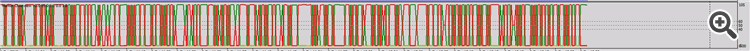But as soon as the first tick comes in it works fine (although the coding itself is "loose" so to speak)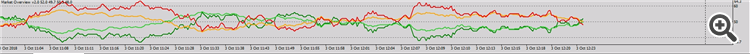I believe the error root cause lies in line 107 which then calls repeat calculations on bars that haven't changed since the previous run of the function thus bogging down the terminal

```   ArrayResize(BullPowerIndicator,Bars+1);
ArrayResize(BearPowerIndicator,Bars+1);

for(int i=0; i<rates_total; i++)
{
Sentiment(i);
Power(i);
```

So I re-engineered the code to try and fix the solution but have come to a dead end.

Here is the new code (hopefully much cleaner than before)

```//+------------------------------------------------------------------+
//|                               Master Indicator Template v3.0 mq4 |
//|                                                forexbob@live.com |
//+------------------------------------------------------------------+
#property version   "3.0"
#property strict
#property indicator_separate_window

//+------------------------------------------------------------------+
//|                                                                  |
//+------------------------------------------------------------------+
//--- indicator settings
#property indicator_separate_window
#property indicator_buffers 4
#property indicator_color1 Green
#property indicator_color2 Red
#property indicator_color3 LimeGreen
#property indicator_color4 Magenta
//--- indicator levels
#property indicator_level1     40.0
#property indicator_level2     50.0
#property indicator_level3     60.0
#property indicator_levelcolor clrDimGray
#property indicator_levelstyle STYLE_DOT

//--- input parameter
input int    InpPeriod=120;

double   BullSentimentIndicator[],BearSentimentIndicator[],BullPowerIndicator[],BearPowerIndicator[];//--- Indicator buffers
double   Body[],Range[],BullBodySize[],Sentiment[],SentimentCount[],BullBodyCount[],TotalPipCount[];//--- internal count buffers
//+------------------------------------------------------------------+
//| Custom indicator initialization function                         |
//+------------------------------------------------------------------+
int OnInit(void)
{
string short_name;

//--- 7 additional buffer used for counting.
IndicatorBuffers(11);
SetIndexBuffer(0,BullPowerIndicator);   //--- indicator buffer for display
SetIndexBuffer(1,BearPowerIndicator);   //--- indicator buffer for display
SetIndexBuffer(2,BullSentimentIndicator);   //--- indicator buffer for display
SetIndexBuffer(3,BearSentimentIndicator);   //--- indicator buffer for display
SetIndexBuffer(4,Body);   //--- internal array to store body size  of each candle
SetIndexBuffer(5,Range);  //--- internal array to store true range of each candle
SetIndexBuffer(6,BullBodySize);   //--- internal array to store (if bull candle) body size  of each candle
SetIndexBuffer(7,Sentiment);  //--- internal array to store sentiment calculation of each candle
SetIndexBuffer(8,SentimentCount);  //--- internal array to store the rolling sentiment sum for input period
SetIndexBuffer(9,BullBodyCount);  //--- internal array to store the rolling Bull body sum for input period
SetIndexBuffer(10,TotalPipCount);  //--- internal array to store the rolling Total body sum for input period

//--- Accuracy of Indicator
IndicatorDigits(1);

//--- drawing style
SetIndexStyle(0,DRAW_LINE,STYLE_SOLID,2);
SetIndexStyle(1,DRAW_LINE,STYLE_SOLID,2);
SetIndexStyle(2,DRAW_LINE,STYLE_SOLID,2);
SetIndexStyle(3,DRAW_LINE,STYLE_SOLID,2);

//--- name for DataWindow and indicator subwindow label
short_name="Bobs Strength Meter("+IntegerToString(InpPeriod)+")";

SetIndexLabel(1,"Sell Power");
SetIndexLabel(3,"Sell Body");

//--- check for input parameter
if(InpPeriod<=0)
{
Print("Wrong input parameter Delta = ",InpPeriod);
return(INIT_FAILED);
}
//---
SetIndexDrawBegin(0,InpPeriod);
//---
return(INIT_SUCCEEDED);
}
//+------------------------------------------------------------------+
//|                                                |
//+------------------------------------------------------------------+
int OnCalculate(const int rates_total,
const int prev_calculated,
const datetime &time[],
const double &open[],
const double &high[],
const double &low[],
const double &close[],
const long &tick_volume[],
const long &volume[],
{
int i,limit;

//--- check for bars count and input parameter
if(rates_total<=InpPeriod || InpPeriod<=0)
return(0);

//--- counting from 0 to rates_total
ArraySetAsSeries(BullPowerIndicator,false);
ArraySetAsSeries(BearPowerIndicator,false);
ArraySetAsSeries(BullSentimentIndicator,false);
ArraySetAsSeries(BearSentimentIndicator,false);
ArraySetAsSeries(Body,false);
ArraySetAsSeries(Range,false);
ArraySetAsSeries(Sentiment,false);
ArraySetAsSeries(Sentiment,false);
ArraySetAsSeries(SentimentCount,false);
ArraySetAsSeries(BullBodyCount,false);
ArraySetAsSeries(TotalPipCount,false);
ArraySetAsSeries(open,false);
ArraySetAsSeries(high,false);
ArraySetAsSeries(low,false);
ArraySetAsSeries(close,false);

//--- preliminary calculations
if(prev_calculated==0)
{
BullPowerIndicator=0.0;
BearPowerIndicator=0.0;
BullSentimentIndicator=0.0;
BearSentimentIndicator=0.0;
Body=0.0;
Range=0.0;
Sentiment=0.0;
SentimentCount=0.0;
BullBodySize=0.0;
BullBodyCount=0.0;
TotalPipCount=0.0;

//--- filling out the array of count values for each period
for(i=1; i<rates_total; i++)
{
bool   IsBullBar=close[i]>open[i];   //--- To determine if current candle direction

Body[i]      = MathAbs(close[i]-open[i]);   //--- Calculate the body size
Range[i]     = MathMax(high[i],close[i-1])-MathMin(low[i],close[i-1]);   //--- Calculate the true range
Sentiment[i] = (Body[i]/Range[i])*100; //--- Sentiment strength is a Percentage calculation body vs range.

if(IsBullBar)  //--- if the current bar is a bull bar assign body to array. A bear bar has the value of 0 for counting purposes.
BullBodySize[i]=Body[i];
else
BullBodySize[i]=0;
}

//--- first Period values of the indicator are not calculated
double MovingSentimentCount=0.0;  //--- variable to store the accumulated sentiment count for the input period
double MovingBullBodyCount =0.0;  //--- variable to store the accumulated bull body count for the input period
double MovingPipcount=0.0;   //--- variable to store the accumulated range for the input period

for(i=1; i<=InpPeriod; i++)
{
BullPowerIndicator[i] = 0.0;
BearPowerIndicator[i] = 0.0;
BullPowerIndicator[i] = 0.0;
BearPowerIndicator[i] = 0.0;

MovingSentimentCount+=Sentiment[i];
MovingBullBodyCount+=BullBodySize[i];
MovingPipcount+=Range[i];
}

//--- calculating the first value of the indicator
BullPowerIndicator[InpPeriod] = MovingSentimentCount/InpPeriod;
BearPowerIndicator[InpPeriod] = 100-(MovingSentimentCount/InpPeriod);
BullSentimentIndicator[InpPeriod] = (MovingBullBodyCount/MovingPipcount)*100;
BearSentimentIndicator[InpPeriod] = 100-((MovingBullBodyCount/MovingPipcount)*100);

limit=InpPeriod+1;
}

else
limit=prev_calculated-1;

//--- the main loop of calculations
for(i=limit; i<rates_total; i++)
{
bool   IsBullBar=close[i]>open[i];

Body[i]  = MathAbs(close[i]-open[i]);
Range[i] = MathMax(high[i],close[i-1])-MathMin(low[i],close[i-1]);

if(Body[i]==0 || Range[i]==0)
Sentiment[i]=50;
else
Sentiment[i]=(Body[i]/Range[i])*100;

SentimentCount[i]=SentimentCount[i-1]+Sentiment[i]-Sentiment[i-InpPeriod];

if(IsBullBar)
BullBodySize[i]=Body[i];
else
BullBodySize[i]=0;

BullBodyCount[i]=BullBodyCount[i-1]+Body[i]-Body[i-InpPeriod];

TotalPipCount[i]=TotalPipCount[i-1]+Range[i]-Range[i-InpPeriod];

//--- calculating the  value of the indicator
BullPowerIndicator[i] = SentimentCount[i]/InpPeriod;
BearPowerIndicator[i] = 100 - BullPowerIndicator[InpPeriod];
BullPowerIndicator[i] = (BullBodyCount[i]/TotalPipCount[i])*100;
BearPowerIndicator[i] = 100-((BullBodyCount[i]/TotalPipCount[i]))*100;
}
return(rates_total);
}```

But when I apply to my chart all I get is this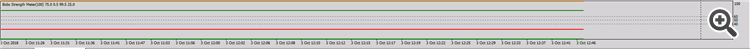My journal tab says it loads successfully and is initialized ans so to the expert tab.

Any help getting this one to work will be greatly appreciated.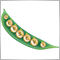7151

A few of the buffer names are duplicated in the calculations and one in ArraySetAsSeries().127

Ernst Van Der Merwe:
A few of the buffer names are duplicated in the calculations and one in ArraySetAsSeries().

So I looked at it and thought about the code, changed this

```//--- preliminary calculations
if(prev_calculated==0)
{
BullPowerIndicator=0.0;
BearPowerIndicator=0.0;
BullSentimentIndicator=0.0;
BearSentimentIndicator=0.0;
Body=0.0;
Range=0.0;
Sentiment=0.0;
SentimentCount=0.0;
BullBodySize=0.0;
BullBodyCount=0.0;
TotalPipCount=0.0;

//--- filling out the array of count values for each period
for(i=1; i<rates_total; i++)
{
bool   IsBullBar=close[i]>open[i];   //--- To determine if current candle direction

Body[i]      = MathAbs(close[i]-open[i]);   //--- Calculate the body size
Range[i]     = MathMax(high[i],close[i-1])-MathMin(low[i],close[i-1]);   //--- Calculate the true range
Sentiment[i] = (Body[i]/Range[i])*100; //--- Sentiment strength is a Percentage calculation body vs range.

if(IsBullBar)  //--- if the current bar is a bull bar assign body to array. A bear bar has the value of 0 for counting purposes.
BullBodySize[i]=Body[i];
else
BullBodySize[i]=0;
}

//--- first Period values of the indicator are not calculated
double MovingSentimentCount=0.0;  //--- variable to store the accumulated sentiment count for the input period
double MovingBullBodyCount =0.0;  //--- variable to store the accumulated bull body count for the input period
double MovingPipcount=0.0;   //--- variable to store the accumulated range for the input period

for(i=1; i<=InpPeriod; i++)
{
BullPowerIndicator[i] = 0.0;
BearPowerIndicator[i] = 0.0;
BullPowerIndicator[i] = 0.0;
BearPowerIndicator[i] = 0.0;

MovingSentimentCount+=Sentiment[i];
MovingBullBodyCount+=BullBodySize[i];
MovingPipcount+=Range[i];
}

//--- calculating the first value of the indicator
BullPowerIndicator[InpPeriod] = MovingSentimentCount/InpPeriod;
BearPowerIndicator[InpPeriod] = 100-(MovingSentimentCount/InpPeriod);
BullSentimentIndicator[InpPeriod] = (MovingBullBodyCount/MovingPipcount)*100;
BearSentimentIndicator[InpPeriod] = 100-((MovingBullBodyCount/MovingPipcount)*100);

limit=InpPeriod+1;
}

else
limit=prev_calculated-1;

//--- the main loop of calculations
for(i=limit; i<rates_total; i++)
{
bool   IsBullBar=close[i]>open[i];

Body[i]  = MathAbs(close[i]-open[i]);
Range[i] = MathMax(high[i],close[i-1])-MathMin(low[i],close[i-1]);

if(Body[i]==0 || Range[i]==0)
Sentiment[i]=50;
else
Sentiment[i]=(Body[i]/Range[i])*100;

SentimentCount[i]=SentimentCount[i-1]+Sentiment[i]-Sentiment[i-InpPeriod];

if(IsBullBar)
BullBodySize[i]=Body[i];
else
BullBodySize[i]=0;

BullBodyCount[i]=BullBodyCount[i-1]+Body[i]-Body[i-InpPeriod];

TotalPipCount[i]=TotalPipCount[i-1]+Range[i]-Range[i-InpPeriod];

//--- calculating the  value of the indicator
BullPowerIndicator[i] = SentimentCount[i]/InpPeriod;
BearPowerIndicator[i] = 100 - BullPowerIndicator[InpPeriod];
BullPowerIndicator[i] = (BullBodyCount[i]/TotalPipCount[i])*100;
BearPowerIndicator[i] = 100-((BullBodyCount[i]/TotalPipCount[i]))*100;
}```

to this

```      //--- filling out the array of count values for each period
for(i=1; i<=InpPeriod; i++)
{
bool   IsBullBar=close[i]>open[i];   //--- To determine if current candle direction

Body[i]=MathAbs(close[i]-open[i]);   //--- Calculate the body size
Range[i]=MathMax(high[i],close[i-1])-MathMin(low[i],close[i-1]);   //--- Calculate the true range
Sentiment[i]=(Body[i]/Range[i])*100; //--- Sentiment strength is a Percentage calculation body vs range.

if(IsBullBar) //--- if the current bar is a bull bar assign body to array. A bear bar has the value of 0 for counting purposes.
BullBodySize[i]=Body[i];
else
BullBodySize[i]=0;

SentimentCount[i]+=SentimentCount[i-1]+Sentiment[i];
BullBodyCount[i]+=BullBodyCount[i-1]+BullBodySize[i];
TotalPipCount[i]+= TotalPipCount[i-1]+Range[i];

BullPowerIndicator[i] = SentimentCount[i]/i;
BearPowerIndicator[i] = 100-(SentimentCount[i]/i);
BullSentimentIndicator[i] = (BullBodyCount[i]/TotalPipCount[i])*100;
BearSentimentIndicator[i] = 100-((BullBodyCount[i]/TotalPipCount[i])*100);
}

limit=InpPeriod+1;
}

else
limit=prev_calculated-1;

//--- the main loop of calculations
for(i=limit; i<rates_total; i++)
{
bool   IsBullBar=close[i]>open[i];

Body[i]  = MathAbs(close[i]-open[i]);
Range[i] = MathMax(high[i],close[i-1])-MathMin(low[i],close[i-1]);

if(Body[i]==0 || Range[i]==0)
Sentiment[i]=50;
else
Sentiment[i]=(Body[i]/Range[i])*100;

if(IsBullBar)
BullBodySize[i]=Body[i];
else
BullBodySize[i]=0;

SentimentCount[i]=SentimentCount[i-1]+Sentiment[i]-Sentiment[i-InpPeriod];
BullBodyCount[i]=BullBodyCount[i-1]+Body[i]-Body[i-InpPeriod];
TotalPipCount[i]=TotalPipCount[i-1]+Range[i]-Range[i-InpPeriod];

//--- calculating the  value of the indicator
BullPowerIndicator[i] = SentimentCount[i]/InpPeriod;
BearPowerIndicator[i] = 100 - BullPowerIndicator[i];
BullPowerIndicator[i] = (BullBodyCount[i]/TotalPipCount[i])*100;
BearPowerIndicator[i] = 100-BullPowerIndicator[i];
}```

but alais no success

Any other thoughts127

So i filled the code up with a heap of print functions. Pulled up the log files and work through each line.

Found the issues and have corrected them. Now I have a beautiful looking indicator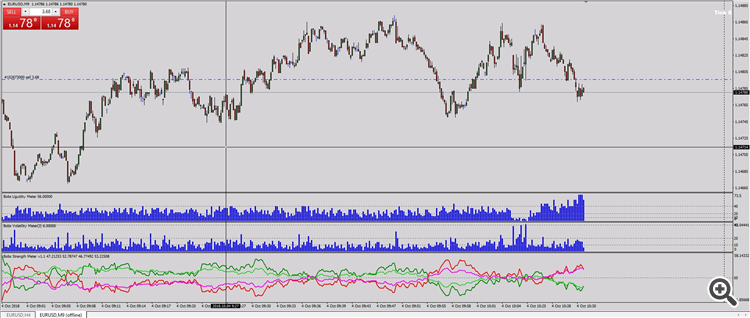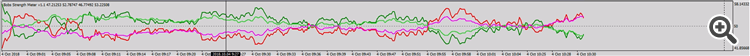Thanks Ernst for responding. Better than others